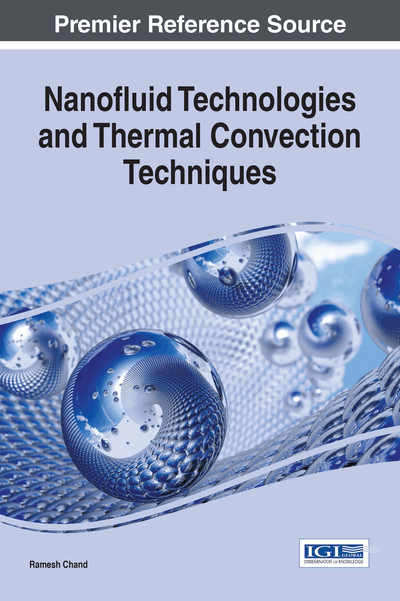# Double Diffusive Convection in a Layer of Maxwellian Visco-Elastic Nanofluid in a Porous Medium

Copyright: © 2017 |Pages: 20
DOI: 10.4018/978-1-68318-006-7.ch011

Top

## Mathematical Formulations Of The Problem

### The Physical Problem

The physical configuration of the problem to be considered as An infinite horizontal layer of Maxwellian visco-elastic nanofluid in a porous medium of medium permeability k1 and porosity ε and of thickness ‘d’ bounded by horizontal boundaries z = 0 and z = d. A Cartesian coordinate system (x, y, z) is chosen with the origin at the bottom of the fluid layer and the z- axis normal to the fluid layer. Fluid layer is acted upon by gravity force g(0, 0,-g). Fluid layer is heated from below in such a way that horizontal boundaries z = 0 and z = d respectively maintained at a uniform temperature T0 and T1 (T0 > T1). The normal component of the nanoparticles flux has to vanish at an impermeable boundary and the temperature T is taken to be T0 at z = 0 and T1 at z = d, (T0 > T1) as shown in Figure 1. The reference scale for temperature and nanoparticles fraction is taken to be T1 and φ0 respectively.

## Complete Chapter List

Search this Book:
Reset